# LOX Mechanical Design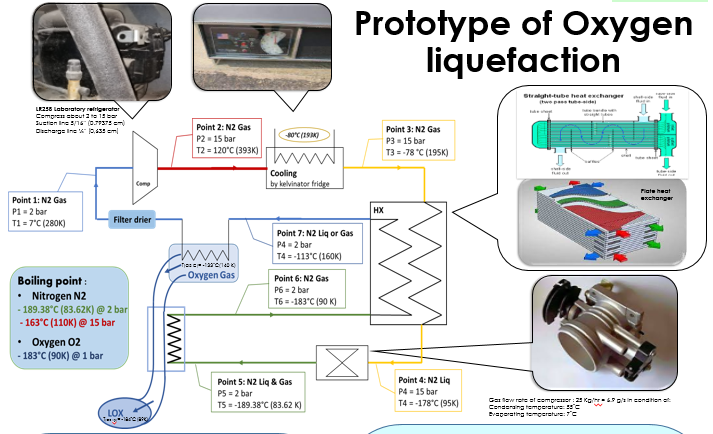Overview: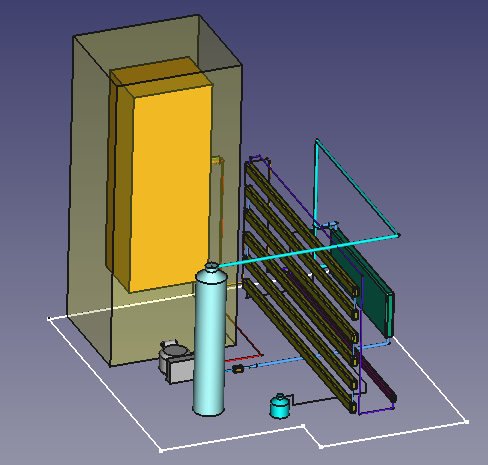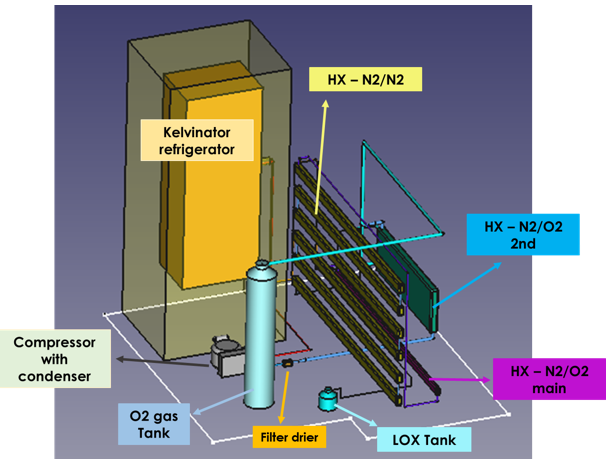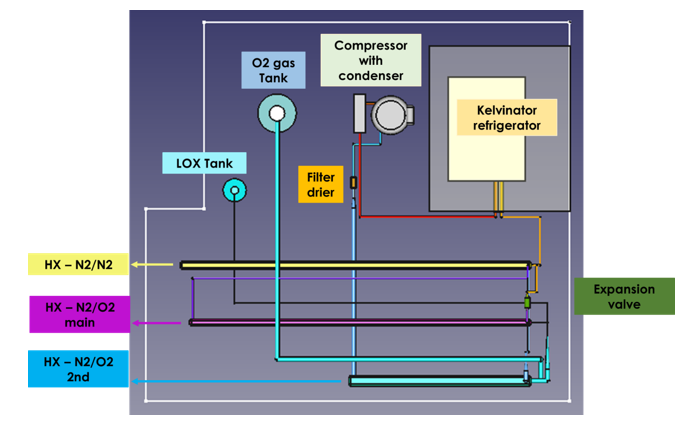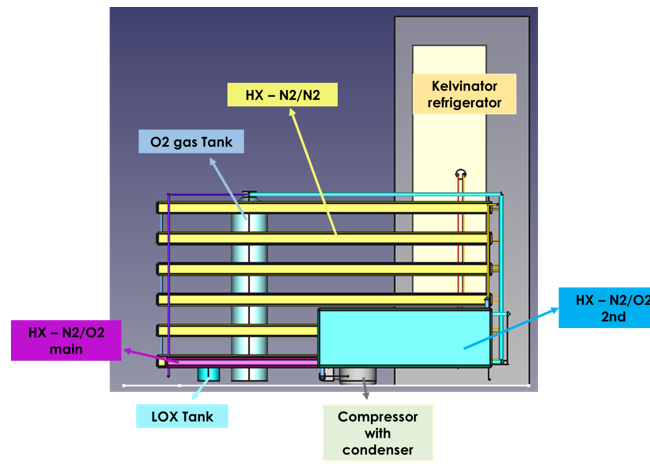Cooler Design:

The cooler is presented by a tunned refrigerator by adding seven cooling tubes inside it.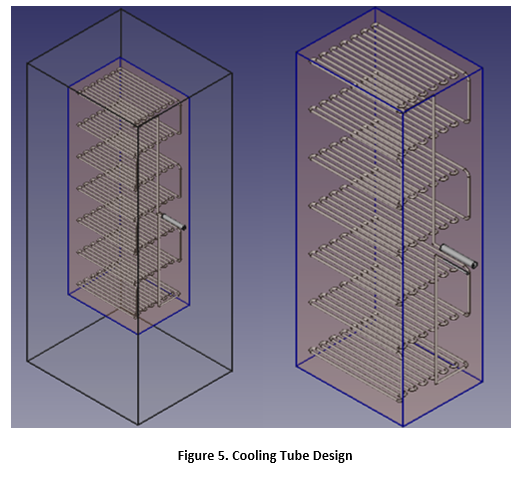310821_ICPT_LOXPrototype(.docx)

• Calculation pressure of pipes

Equation: P = (2*S*T)/((O.D.-2*T)*SF)

Where:

P = Fluid Pressure (psi)

T = Pipe wall thickness (in)

O.D. = Pipe outside diameter (in)

Sf = Safety factor (General Calculations 1.5 – 10, Use 1 For Bursting Pressure)

S = Material strength (psi)

Ultimate Tensile strength or Yield strength can be used.

Ultimate should be used to determine the bursting pressure.

Yield can be used for estimating pressures at which permanent deformation begins.

1Mpa=144.55psi

In our case, We have 2 types of pipes: one made of aluminum (Al) and the other of copper (Cu). In the table below, information about these two types of pipes

 Material Aluminum (Al) Copper (Cu) O.D. [in] 0.3228 0.37874 T [in] 0.037385 0.047244 S [psi] 1450.3774 30457.9262 Sf min 1.5 1.5 Sf max 10 10 P [psi] (Sf min) 655.84 15186.75 P [psi] (Sf max) 4372.24 101244.97 P [bar] (Sf min) 45.22 1047.09 P [bar] (Sf max) 301.46 6980.60

# Aluminum Pipe Equations Formulas Design Calculator 

 https://www.ajdesigner.com/phpaluminumpipe/pressure_rating_equation.php#ajscroll

# Solving for pressure rating

##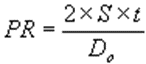`Note: Under most cases, S = 7500 pound/inch2 for aluminum`